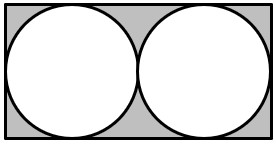Select Page

Each month, a new set of puzzles will be posted.  Come back next month for the solutions and a new set of puzzles, or subscribe to have them sent directly to you.

 How many complete turns does circle A make to rotate around the outside of circle B once if circle A is one-half the size of circle B. (Note: Diagram is not drawn to scale.)How many complete turns does circle A make to rotate around the inside of circle B once if circle A is one-half the size of circle B. (Note: Diagram is not drawn to scale.)To get a square photograph to fit into a rectangular frame, Brian had to trim a 1-inch strip from each of two opposite sides of the photo and a 2-inch strip from each of the other two sides. In all, he trimmed of 64 squire inches. What were the original dimensions of the photograph?

## Feedback

There are more than one way of doing these puzzles and may well be more than one answer.  Please let me and others know what alternatives you find by commenting below.  We also welcome general comments on the subject and any feedback you'd like to give.

If you have a question that needs a response from me or you would like to contact me privately, please use the contact form.

## Get more puzzles!

If you've enjoyed doing the puzzles, consider ordering the books;

• Book One - 150+ of the best puzzles
• Book Two - 200+ with new originals and more of your favourites

Both in a handy pocket sized format.   Click here for full details.

## MIND-Xpanders

1. What is the number of degrees that the hour hand of a clock moves through between noon and 4:30 in the afternoon of the same day?
Solution: 360º / 12 numbers = 30º and 30º x 4.5 numbers = 135º
2. Raeanne takes 20 minutes to jog around the race track course once, and 25 minutes to jog around a second time. What is her average speed in miles per hour for the whole jog if the course is 3 miles long?
Solution:
Average speed = total distance / total time
Total distance covered = 2 x 3 = 6 miles
Total time = 20 + 25 = 45 minutes or 0.75 hours
Average speed = 6 / 0.75 = 8 miles/hour
3. Two equal circles are cut out of a rectangular paper card of dimensions 8 cm by 16 cm. The circles have a maximum radius possible. What is the area of the paper remaining (shadded area) after the circles have been cut out as shown? (note: use π = 3.14)Solution:

The area remaining (or in gray) is the area of the rectangle minus 2 times the area of a circle

= (16 x 8) – 2 x (πr²), where r = 16/4 = 4,
then (128) – (2 x 3.14 x 16)
= 128 – 100.48
= 27.52 cm.